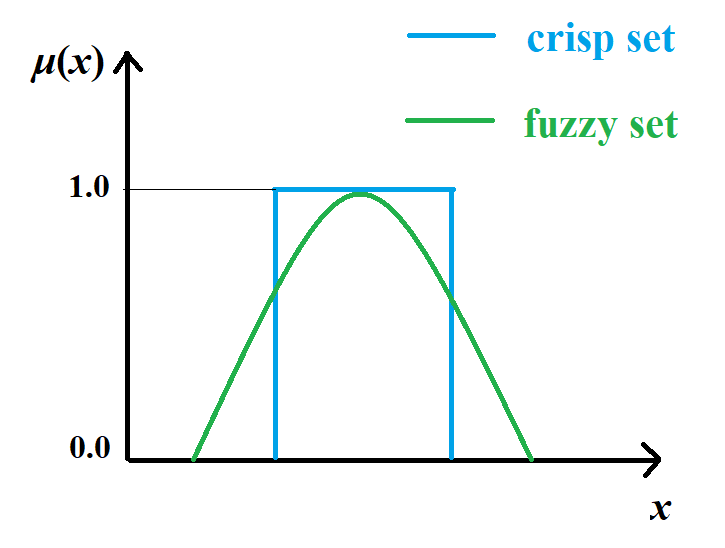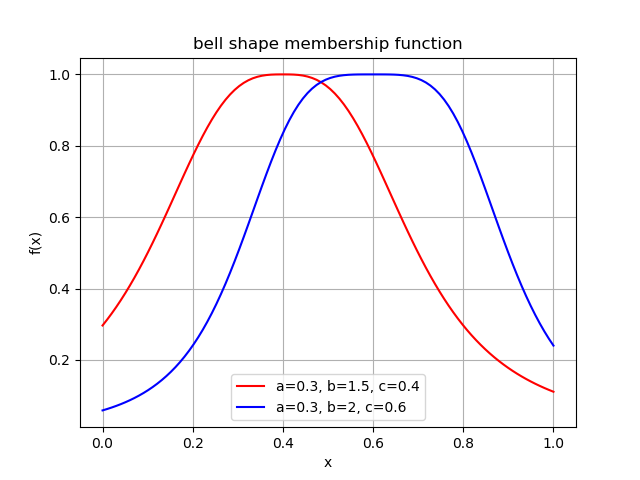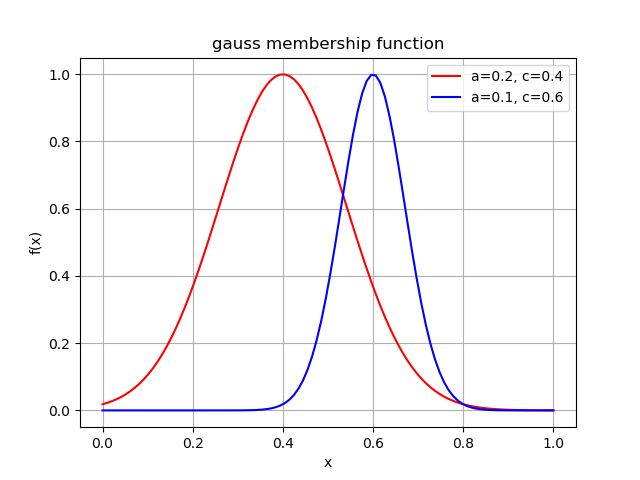# 2.2. 隶属函数¶

## 2.2.1. 什么是隶属函数¶

The membership function of a fuzzy set is a generalization of the indicator function in classical sets. In fuzzy logic, it represents the degree of truth as an extension of valuation. Degrees of truth are often confused with probabilities, although they are conceptually distinct, because fuzzy truth represents membership in vaguely defined sets, not likelihood of some event or condition. Membership functions were introduced by Zadeh in the first paper on fuzzy sets (1965). Zadeh, in his theory of fuzzy sets, proposed using a membership function (with a range covering the interval (0,1)) operating on the domain of all possible values.

Definition 2.12 (隶属函数)Membership Function of crisp set and fuzzy set.

## 2.2.2. 常见隶属函数¶

### 钟形曲线¶

$f(x) = \frac{1}{1 + |\frac{x-c}{a}|^{2b}}$Bell Shape Membership Function.

### 高斯函数¶

$f(x) = {\rm exp}\left(\frac{-\|x-c\|^2}{a^2}\right)$Gauss Membership Function.